# 题目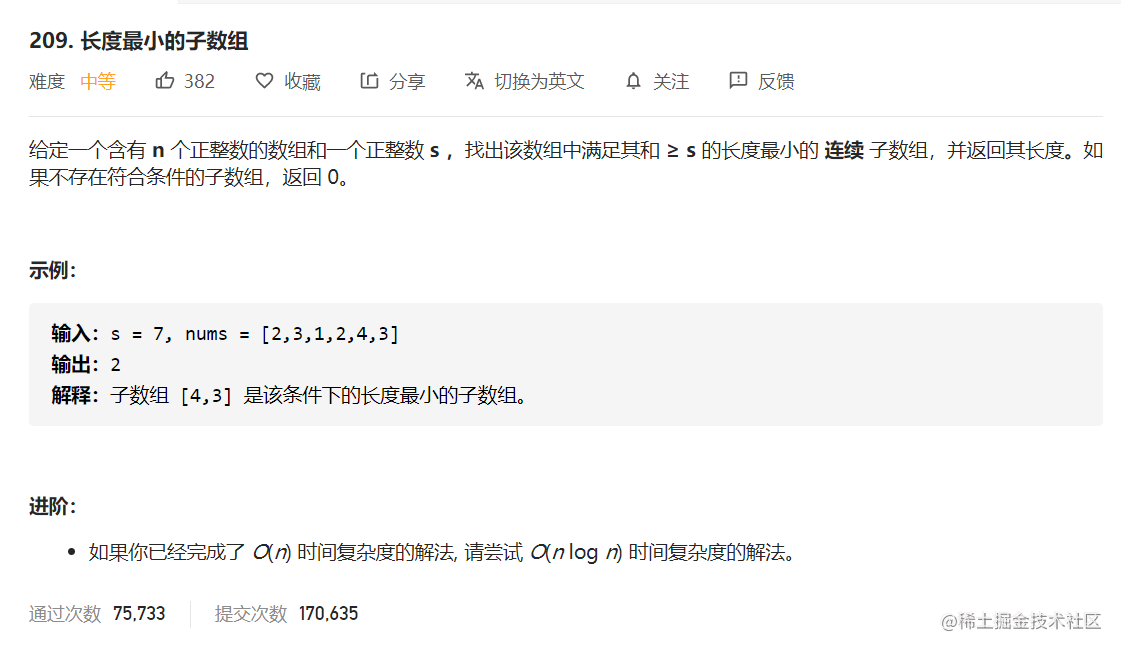##### 1，暴力求解，

``````    public int minSubArrayLen(int s, int[] nums) {
int min = Integer.MAX_VALUE;
for (int i = 0; i < nums.length; i++) {
int sum = nums[i];
if (sum >= s)
return 1;
for (int j = i + 1; j < nums.length; j++) {
sum += nums[j];
if (sum >= s) {
min = Math.min(min, j - i + 1);
break;
}
}
}
return min == Integer.MAX_VALUE ? 0 : min;
}

##### 2，使用队列相加（实际上我们也可以把它称作是滑动窗口，这里的队列其实就相当于一个窗口）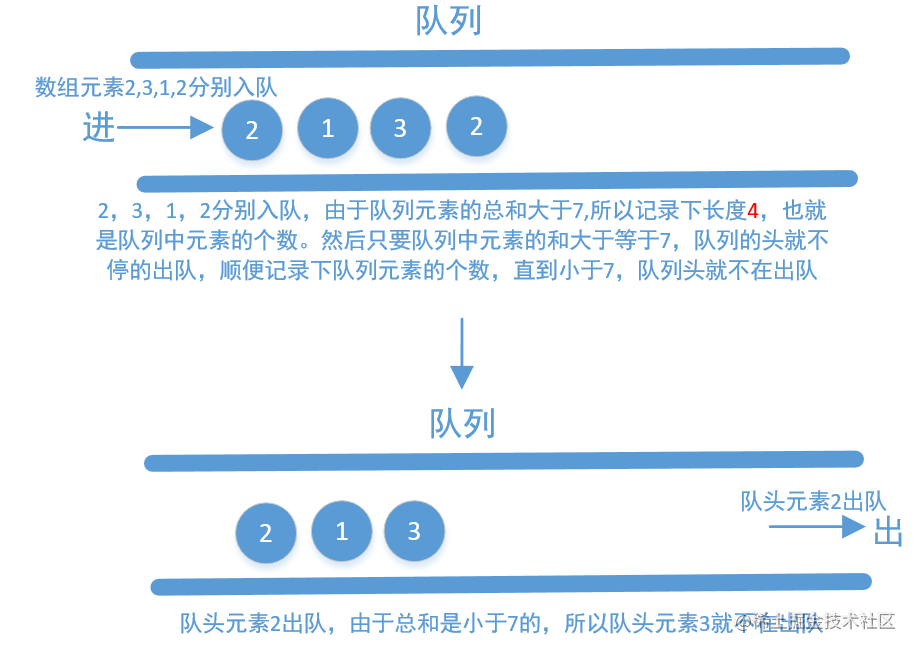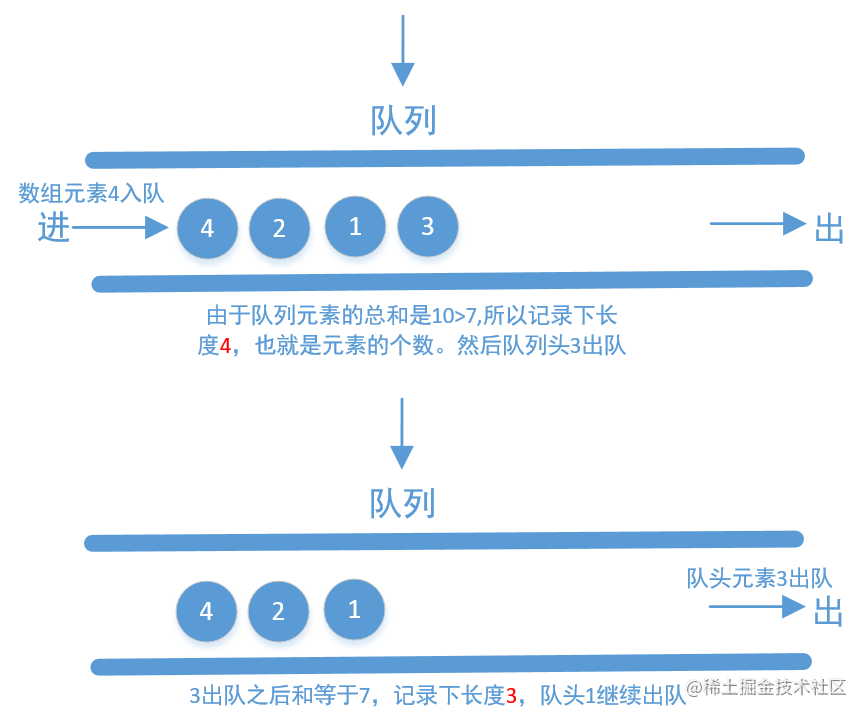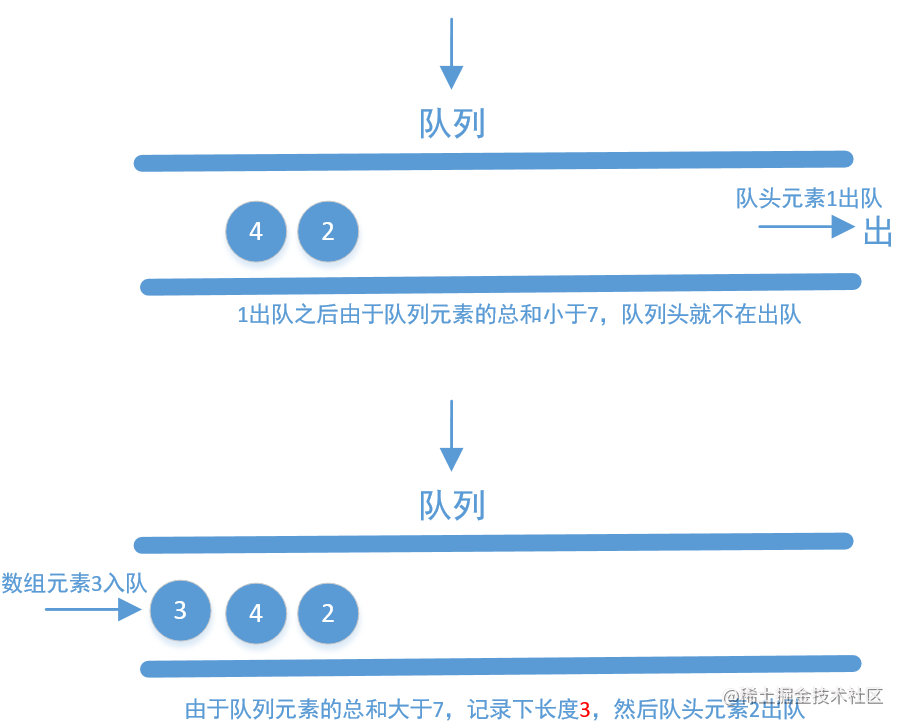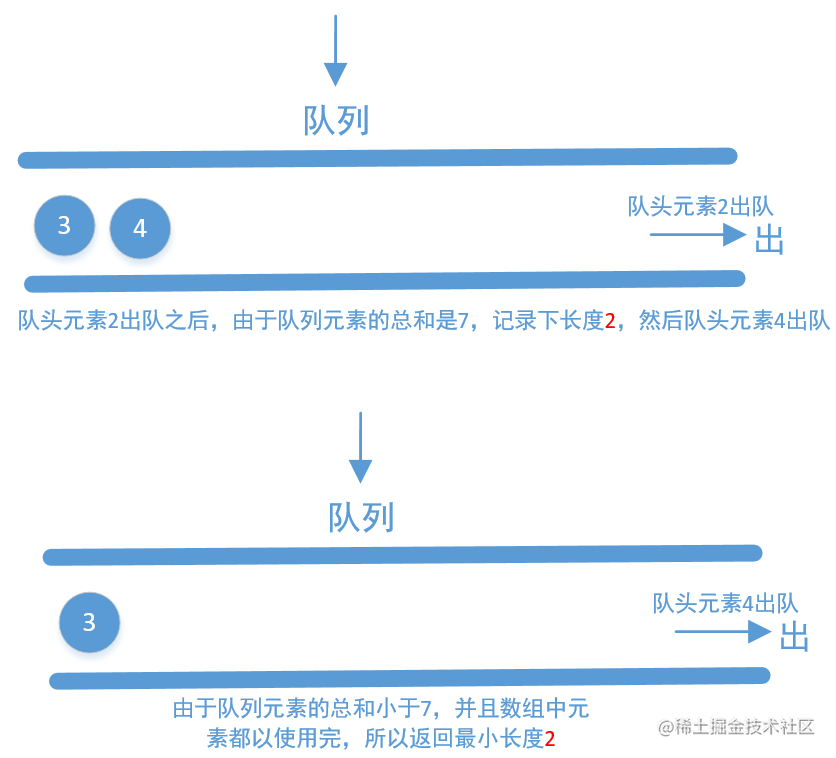``````    public int minSubArrayLen(int s, int[] nums) {
int lo = 0, hi = 0, sum = 0, min = Integer.MAX_VALUE;
while (hi < nums.length) {
sum += nums[hi++];
while (sum >= s) {
min = Math.min(min, hi - lo);
sum -= nums[lo++];
}
}
return min == Integer.MAX_VALUE ? 0 : min;
}##### 3，使用队列相减

``````    public int minSubArrayLen(int s, int[] nums) {
int lo = 0, hi = 0, min = Integer.MAX_VALUE;
while (hi < nums.length) {
s -= nums[hi++];
while (s <= 0) {
min = Math.min(min, hi - lo);
s += nums[lo++];
}
}
return min == Integer.MAX_VALUE ? 0 : min;
}

##### 4，二分法查找

``````    public int minSubArrayLen(int s, int[] nums) {
int length = nums.length;
int min = Integer.MAX_VALUE;
int[] sums = new int[length + 1];
for (int i = 1; i <= length; i++) {
sums[i] = sums[i - 1] + nums[i - 1];
}
for (int i = 0; i <= length; i++) {
int target = s + sums[i];
int index = Arrays.binarySearch(sums, target);
if (index < 0)
index = ~index;
if (index <= length) {
min = Math.min(min, index - i);
}
}
return min == Integer.MAX_VALUE ? 0 : min;
}

##### 5，直接使用窗口

``````    public int minSubArrayLen(int s, int[] nums) {
int lo = 1, hi = nums.length, min = 0;
while (lo <= hi) {
int mid = (lo + hi) >> 1;
if (windowExist(mid, nums, s)) {
hi = mid - 1;//找到就缩小窗口的大小
min = mid;//如果找到就记录下来
} else
lo = mid + 1;//没找到就扩大窗口的大小
}
return min;
}

//size窗口的大小
private boolean windowExist(int size, int[] nums, int s) {
int sum = 0;
for (int i = 0; i < nums.length; i++) {
if (i >= size)
sum -= nums[i - size];
sum += nums[i];
if (sum >= s)
return true;
}
return false;
}
size];
sum += nums[i];
if (sum >= s)
return true;
}
return false;
}